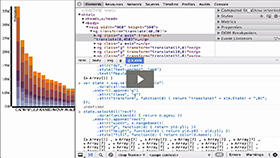Basic Chart - Stacked Bar Chart
Duration: 22:16
Part of Course: Introductory D3 Course
This Lesson is for subscribersUnlock this lesson NOW!
• Get expert advice on Basic Chart - Stacked Bar Chart
• Start mastering D3.js right now!

Takeaways:

• You will use the CSV data from the D3js.org website Stacked Bar Chart Example to see how a full D3 Stacked Bar Chart Example data visualization is built
• Notice that the styling is done in the <style> </style> section of the HTML document
• Notice the D3 Margin Convention
• Notice the D3 Ordinal Scale for the X-Axis and the rangeRoundBands
• Notice the D3 Linear Scale for the Y-Axis
• Notice the D3 Ordinal Scale for the 7 HTML colors used to encode attributes of the data
• Notice the D3 SVG Axis component creation and definition for the X-Axis and Y-Axis
• Notice the D3 .tickFormat to format the ticks on the Y-Axis
• Notice the D3 Type-Specific d3.csv AJAX call
• Notice the use of the d3.keys operator
• Notice the use the of the .filter, .map, and .forEach array functions
• Notice the JavaScript array sorting method
• Notice the .domain being set for the primary X-Axis and Y-Axis
• Notice the D3 Data Join
• Notice the secondary D3 Data Join with a function used to pull out data to bind to the SVG Rectangle DOM Elements
• Notice the Third D3 Data Join for the Grouped Bar Chart Legend
• Notice how D3 helps create visual representations of the data, stacking of data, and the legend

Resources:
This Transcript is for subscribers.Unlock this D3 Tutorial NOW!
• Get expert advice on Basic Chart - Stacked Bar Chart